Next: Uniformly Magnetized Sphere Up: Magnetostatics in Magnetic Media Previous: Boundary Conditions for and

# Permanent Ferromagnets

Let us consider the magnetic field generated by a distribution of permanent ferromagnets. Suppose that the magnets in question are sufficiently hard'' that their magnetization is essentially independent of the applied field for moderate field-strengths. Such magnets can be treated as if they contain a fixed magnetization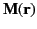.

Let us assume that there are no true currents in the problem, so that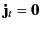. Let us also assume that we are dealing with a steady-state situation. Under these circumstances Equation (688) reduces to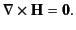(699)

It follows that we can write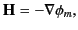(700)

where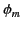is called the magnetic scalar potential. Now, we know that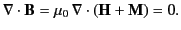(701)

Equations (701) and (702) combine to give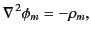(702)

where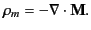(703)

Thus, the magnetostatic field,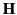, is determined by Poisson's equation. We can think of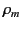as an effective magnetic charge density. Of course, this magnetic charge has no physical reality. We have only introduced it in order to make the problem of the steady magnetic field generated by a set of permanent magnets look formally the same as that of the steady electric field generated by a distribution of charges.

The unique solution of Poisson's equation, subject to sensible boundary conditions at infinity, is well known (see Section 2.3):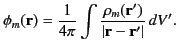(704)

This solution yields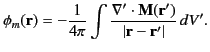(705)

If the magnetization fieldis well behaved and localized then we can integrate by parts to obtain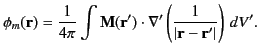(706)

Now,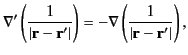(707)

so our expression for the magnetic potential can be written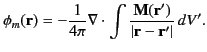(708)

Far from the region of non-vanishing magnetization, the potential reduces to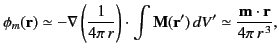(709)

where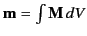is the total magnetic moment of the distribution. This is the scalar potential of a dipole. (See Sections 3.6 and 5.5.) Thus, an arbitrary localized distribution of magnetization asymptotically produces a dipole magnetic field whose strength is determined by the net magnetic moment of the distribution.

It is often a good approximation to treat the magnetization fieldas a discontinuous quantity. In other words,is specified throughout the hard'' ferromagnets in question, and suddenly falls to zero at the boundaries of these magnets. Integrating Equation (704) over a Gaussian pill-box that straddles one of these boundaries leads to the conclusion that there is an effective magnetic surface charge density,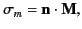(710)

on the surface of the ferromagnets, where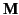is the surface magnetization, and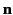is a unit outward directed normal to the surface. Under these circumstances, Equation (706) yields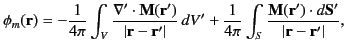(711)

where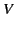represents the volume occupied by the magnets and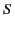is the bounding surface to. Here,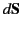is an outward directed element of. It is clear that the right-hand side of Equation (712) consists of a volume integral involving the volume magnetic charges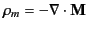, and a surface integral involving the surface magnetic charges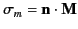. If the magnetization is uniform throughout the volumethen the volume integral vanishes, and only the surface integral makes a contribution.

We can also writein order to satisfy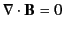automatically. It follows from Equations (688) and (689) that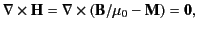(712)

which gives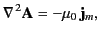(713)

because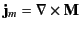. The unique solution to Equation (714), subject to sensible boundary conditions at infinity, is well known: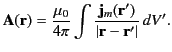(714)

Thus,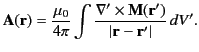(715)

If the magnetization field is discontinuous, it is necessary to add a surface integral to the previous expression. It is straightforward to show that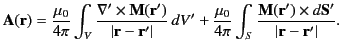(716)

It is clear that the previous expression consists of a volume integral involving the volume magnetization currents, and a surface integral involving the surface magnetization currents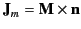[see Equation (699)]. However, if the magnetization field is uniform throughoutthen only the surface integral makes a contribution.Next: Uniformly Magnetized Sphere Up: Magnetostatics in Magnetic Media Previous: Boundary Conditions for and
Richard Fitzpatrick 2014-06-27